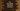# C++ fdim, fdimf and fdiml functions example# fdim, fdimf and fdiml functions in C++ :

fdim, fdimf and fdiml functions are defined in the cmath header. These functions are used to find the difference between two numbers.

They are defined as below :

``````double fdim(double num1, double num2)
float fdimf(float num1, float num2)
long double fdiml(long double num1, long double num2)``````

As you can see, all these functions take two parameters. The return value is double, float or long double. It returns the value of num1 - num2 if num1 > num2. Else, it returns zero.

## Example program :

``````#include <iostream>
#include <cmath>
using namespace std;
int main()
{
float first;
float second;
cout << "Enter the first number : " << endl;
cin >> first;
cout << "Enter the second number : " << endl;
cin >> second;
cout << "fdim : " << fdim(first, second) << endl;
return 0;
}``````

### Sample Output :

``````Enter the first number :
12.44
Enter the second number :
1.3
fdim : 11.14

Enter the first number :
1.3
Enter the second number :
12.44
fdim : 0``````

This is an example of fdim. You can similarly implement for fdimf and fdiml.